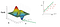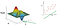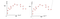# ML Fundamentals: Optimization problems and how to solve them

If you start to look into machine learning and the math behind it, you will quickly notice that everything comes down to an optimization problem. Even the training of neural networks is basically just finding the optimal parameter configuration for a really high dimensional function. So to start understanding Machine Learning algorithms, you need to understand the fundamental concept of mathematical optimization and why it is useful.

In this article, we will go through the steps of solving a simple Machine Learning problem step by step. We will see why and how it always comes down to an optimization problem, which parameters are optimized and how we compute the optimal value in the end.

# Step 1: Problem definition

To start, let’s have a look at a simple dataset (x1, x2):

This dataset can represent whatever we want, like x1 = Age of your computer, x2 = time you need to train a Neural Network for example. Every red dot on our plot represents a measured data point. This plot here represents the ground truth: All these points are correct and known data entries. The problem is that the ground truth is often limited: We know for 11 computer-ages (x1) the corresponding time they needed to train a NN. But what about your computer? If you are lucky, one computer in the dataset had the exactly same age as your, but that’s highly unlikely. For your computer, you know the age x1, but you don’t know the NN training time x2.

# Step 2: Making guesses (the stupid way)

So let’s have a look at a way to solve this problem. Let’s just look at the dataset and pick the computer with the most similar age. If we are lucky, there is a PC with comparable age nearby, so taking the nearby computer’s NN training time will give a good estimation of our own computers training time — e.g. the error we make in guessing the value x2 (training time) will be quite small.

But what if we are less lucky and there is no computer nearby? Then, the error gets extremely large.

We obviously need a better algorithm to solve problems like that.

# Step 3: Making guesses the intelligent, Machine Learning way

## a. Data approximation

Now we enter the field of Machine Learning. If you have a look at the red datapoints, you can easily see a linear trend: The older your PC (higher x1), the longer the training time (higher x2). A better algorithm would look at the data, identify this trend and make a better prediction for our computer with a smaller error.

The grey line indicates the linear data trend. Given an x1 value we don’t know yet, we can just look where x1 intersects with the grey approximation line and use this intersection point as a prediction for x2.

This principle is known as data approximation: We want to find a function, in our case a linear function describing a line, that fits our data as good as possible. We can also say that our function should approximate our data.

## b. Approximation line

But how would we find such a line? First, let’s go back to high-school and see how a line is defined:

In this equation, a defines the slope of our line (higher a = steeper line), and b defines the point where the line crosses the y axis. (Note that the axis in our graphs are called (x1, x2) and not (x, y) like you are used to from school. Don’t be bothered by that too much, we will use the (x, y) notation for the linear case now, but will later come back to the (x1, x2) notation for higher order approximations). To find a line that fits our data perfectly, we have to find the optimal values for both a and b.

For our example data here, we have optimal values a=0.8 and b=20. But how should we find these values a and b?

## c. Finding the parameters (a, b) that minimize the squared error

Well, as we said earlier, we want to find a and b such that the line y=ax+b fits our data as good as possible. Or, mathematically speaking, the error / distance between the points in our dataset and the line should be minimal.

The error for a single point (marked in green) can is the difference between the points real y value, and the y-value our grey approximation line predicted: f(x). It can be calculates as follows:

Here, f is the function f(x)=ax+b representing our approximation line. xi is the points x1 coordnate, yi is the points x2 coordinate. Remember the parameters a=0.8 and b=20? Let’s set them into our function and calculate the error for the green point at coordinates (x1, x2) = (100, 120):

Error = f(x) — yi
Error = f(100) — 120
Error = a*100+b — 120
Error = 0.8*100+20–120
Error = -12

We can see that our approximation line is 12 units too low for this point. To evaluate how good our approximation line is overall for the whole dataset, let’s calculate the error for all points. How can we do this?

Well, first, let’s square the individual errors. This has two reasons:

1. By squaring the errors, we get absolute values (-12->squared->144)
2. With squaring the errors, we get a much higher value for points that are far away from the approximation line. Therefore, if our approximation line misses some points by a far distance, the resulting error will be quite large.

Then, let’s sum up the errors to get an estimate of the overall error:

Or, more generally written:

This formula is called the “Sum of Squared Errors” and it is really popular in both Machine Learning and Statistics.

## d. The optimization function

How is this useful? Well, let’s remember our original problem definition: We want to find a and b such that the linear approximation line y=ax+b fits our data best. Let’s say this with other words: We want to find a and b such that the squared error is minimized.

Tadaa, we have a minimization problem definition. We want to find values for a and b such that the squared error is minimized.

If we find the minimum of this function f(a, b), we have found our optimal a and b values:

Before we get into actual calculations, let’s give a graphical impression of how our optimization function f(a, b) looks like:

Note that the graph on the left is not actually the representation of our function f(a,b), but it looks similar. The height of the landscape represents the Squared error. The higher the mountains, the worse the error. So the minimum squared error is right where our green arrow points to. When we reed out the values for a and b at this point, we get a-optimal and b-optimal.

Going more into the direction of a (e.g. having higher values for a) would give us a higher slope, and therefore a worse error.Example of how the approximation line changes if we change ‘a’

If we went into the direction of b (e.g. having higher values for b), we would shift our line upwards or downwards, giving us worse squared errors as well.Example of how the approximation line changes if we change ‘b’

## e. Calculate the optimal value

So the optimal point indeed is the minimum of f(a,b). But how do we calculate it? Well, we know that a global minimum has to fulfill two conditions:

f’(a,b) = 0 — The first derivative must be zero
f’’(a,b) >0 — The second derivative must be positive

Let’s focus on the first derivative and only use the second one as a validation. Since we have a two-dimensional function, we can simply calculate the two partial derivatives for each dimension and get a system of equations:

f(a, b) Δa = 0
f(a, b) Δb = 0

Let’s rewrite f(a,b) = SUM [axi+b — yi]² by resolving the square. This leaves us with f(a,b) = SUM [yi² + b²+a²x + 2abxi — 2byi — 2bxiyi]. Let’s fill that into our derivatives:

f(a,b) = SUM [yi² + b²+a²x + 2abxi — 2byi — 2axiyi] Δa = 0
f(a,b) = SUM [yi² + b²+a²x + 2abxi — 2byi — 2axiyi] Δb = 0

We can easily calculate the partial derivatives:

f(a,b) = SUM [2ax + 2bxi — 2xiyi] = 0
f(a,b) = SUM [2b+ 2axi — 2yi ] = 0

We can not solve one equation for a, then set this result into the other equation which will then only be dependent on b alone to find b. Finally, we fill the value for b into one of our equal equations to get a.

Why don’t we do that by hand here? Well, remember we have a sum in our equations, and many known values xi and yi. Even for just 10 datapoints, the equation gets quite long. We can let a computer solve it with no problem, but can barely do it by hand.

Congratulations! You now understand how linear regression works and could — in theory — calculate a linear approximation line by yourself without the help of a calculator!

But wait, there’s more

# Approximating polynomials

What if our data didn’t show a linear trend, but a curved one? Like the curve of a squared function?

Well, in this case, our regression line would not be a good approximation for the underlying datapoints, so we need to find a higher order function — like a square function — that approximates our data.

These approximation lines are then not linear approximation, but polynomial approximation, where the polynomial indicates that we deal with a squared function, a cubic function or even a higher order polynomial approximation.

The principle to calculate these is exactly the same, so let me go over it quickly with using a squared approximation function. First, we again define our problem definition: We want a squared function y = ax² + bx + c that fits our data best.

As you can see, we now have three values to find: a, b and c. Therefore, our minimization problem changes slightly as well. While the sum of squared errors is still defined the same way:

Writing it out shows that we now have an optimization function in three variables, a,b and c:

From here on, you continue exactly the same way as shown above for the linear interpolation.

# Overfitting

One question remains: For a linear problem, we could also have used a squared approximation function. Why? Well, with the approximation function y = ax² + bx + c and a value a=0, we are left with y = bx + c, which defines a line that could perfectly fit our data as well. So why not just take a very high order approximation function for our data to get the best result?

Well, we could do that actually. The higher order functions we would choose, the smaller the squared error would be. In fact, if we choose the order of the approximation function to be one less than the number of datapoints we totally have, our approximation function would even go through every single one of our points, making the squared error zero. Perfect, right?

Well, not so much. It is easiest explained by the following picture:Demonstration of overfitting using a very high degree polynomial for approximation

On the left, we have approximated our data with a squared approximation function. On the right, we used an approximation function of degree 10, so close to the total number of data, which is 14.

You see that our approximation function makes strange movements and tries to touch most of the datapoints, but it misses the overall trend of the data. Since it is a high order polynomial, it will completely skyrock for all values greater than the highest datapoint and probably also deliver less reliable results for the intermediate points. So we should have a personal look at the data first, decide what order polynomial will most probably fit best, and then choose an appropriate polynomial for our approximation.

If you are interested in more Machine Learning stories like that, check out my other medium posts!

Written by